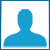330 photos   6617 visits

# Remember _ HowWeMet

Still remember how we met? (: ♥
I mean. I do remember. ♥
But I want you to remember too. ♥
I hope you do, `cuz I really love you. ♥

- Chanel . ♥ -

Name:DeduxaaLov3 20 August 2011
Well nsh exact ..:))) Da tot pe deget`net ne`am imprietenit ..mai tii minte knd te`am asteptat 3 ore in fata la Afi sa vii sa ne vedem ??? x(
xDulceLocuraxD 16 August 2011
i don't :]]] but you ??
xVintageSwagxD 20 August 2011
no=))
yeah. I made this account and you sent me a message "Heeeey. >:D<" and our friendship started & now we're together =))).
xVintageSwagxD 12 August 2011
It`s like a movie=))))))))))))))))))))))))))))))))))))))))))))))))))))
ikr!!! =)))))))))
ColeSprouse 12 August 2011
congrats guys, forgot to mention ;;)
xVintageSwagxD 12 August 2011
thank you Cole. >:D< =))
ColeSprouse 12 August 2011
=))
ColeSprouse 12 August 2011
yeah , when we were sending lots of idiot messages lmao :)
xVintageSwagxD 12 August 2011
=))))))))))))))))))))) No I didn`t . It was Emma=))
ColeSprouse 12 August 2011
whatever =))))
xVintageSwagxD 12 August 2011
" It`s your desicion. You can choose Emma. No, wait. Choose me " =)))))))))))))))))))))))))))))))))))))))))))))))))
ColeSprouse 12 August 2011
omg =))))))))))))))))))))))))))))))))))))))))))))
xVintageSwagxD 12 August 2011
I miss the old times =))))
crazy times. <3 =)))
ColeSprouse 12 August 2011
xCherryShakexD sent a message at 01 Jan 2011, 15:54
Well .. I still catch a grenade for ya` .. sorry I like Bruno Mars .. he looks like Mario LOpezz =P~
xVintageSwagxD 12 August 2011
=)))))))))))))))))))))))))))))))))))))))))))))))))))))))))))))))))))))))))))))))))))))))))) KILL ME !!!!
ColeSprouse 12 August 2011
=)))))))))
ColeSprouse 12 August 2011
xCherryShakexD sent a message at 01 Jan 2011, 15:46
uhm ... I will answer u w/ other question [omg I always wanted to say this :))] .. so .. are u still w/ cleo ?? .
omg =)))))))))))))))
xVintageSwagxD 12 August 2011
ColeSprouse sent a message at 21 Nov 2010, 12:05 [reply] [delete]
:O.Which girl? LoL. The truth is that I've received more messages like these but I never answered them :| I'll never do that to u ;)

.. lol .. Touche =)))))))))))))))))))))))
ColeSprouse 12 August 2011
shut upp =)))))))))))))))))))
xVintageSwagxD 12 August 2011
xlifeincolors.deget.net/ =))))))))))))))))))))))))))))))))))))))))))))))))))))))))))))))))))))))))))))))))))))))))))))))))))))))))))))))))))))))))))
xVintageSwagxD 12 August 2011
YOU shut up =)))))
ColeSprouse 12 August 2011
omg .. that girl told me that all the girls from here are ugly and she is the most beautiful girl in the world =)))))))))
xVintageSwagxD 12 August 2011
ColeSprouse 12 August 2011
=)))))) I think I have more than 30 messages from u talking about Bruno Mars :)))))))))))))))))))))
xVintageSwagxD 12 August 2011
=))))))))))))))))))))))))))))))))))))))))))))))))
ColeSprouse sent a message at 06 Dec 2010, 23:12 [reply] [delete]
:o :o :o . I just found what [ SwaggaCatta.deget] told u, she told me the truth . Gosh. . . she just lied you, you can ask her :|

FAIL. =)))))))))))))))))))))))))))))))))))))))))
xVintageSwagxD 12 August 2011
ColeSprouse sent a message at 04 Dec 2010, 22:54 [reply] [delete]
IDK....what happen....it's over...i mean...don't be mad on me...but i need a lil' while to be alone...:(
ColeSprouse sent a message at 04 Dec 2010, 22:53 [reply] [delete]
Why don't u tell me??:( Are u mad on me?!Tell me please! I won't tell nobody!!!

ColeSprouse 12 August 2011
=)) i told you, idiot messages =)))
xVintageSwagxD 12 August 2011
" I won`t tell nobody " =)))))))))))))))))))))))))))))))))))))
I`m not pregnant =)))
ColeSprouse 12 August 2011
lol =))))))))))))
xVintageSwagxD 12 August 2011
ColeSprouse sent a message at 22 Dec 2010, 17:37 [reply] [delete]
Cleo's not a fake ..:|
ColeSprouse sent a message at 22 Dec 2010, 17:33 [reply] [delete]
Uhm .. I really don't wanna hurt u. :(

who`s laughing noooooooooooooooooooooooow? =))
ColeSprouse 12 August 2011
pleeeeeeeeeeeeeeease shut up =))))))))))))))))))))))))))) :-\$
xVintageSwagxD 12 August 2011
and Lizz... and SwaggaCatta and .. other emo girl?=)))))))))))))))))))))))))))))))))))))
whacha` say now?=))))))))))))))))))))))))))))
ColeSprouse 12 August 2011
xCherryShakexD sent a message at 03 Jan 2011, 20:35
I tald u . .I`m back to Bieber . .. and maybe some Bruno Mars .. :>

=))))))))))))))))))))))))) >:)
xVintageSwagxD 12 August 2011
=)))))))))))))))))))))))))
ColeSprouse sent a message at 03 Jan 2011, 21:22 [reply] [delete]
Well.ok. But it's stupid.:]]] Now some weeks ago, u told me that u love me:| and I've wanted to ask u if.. we can be 2gether again' .But that's idiot. forget it cos' it doesn't matter anymore .:|:-j .

Toucheee. I`m the best =)))))))))))))))))))))))
Read and cry my little Cole >:D< =))
ColeSprouse 12 August 2011
really now
shut up :o3 =))))))))))))))))))))))))))))
xVintageSwagxD 12 August 2011
ColeSprouse sent a message at 10 Dec 2010, 20:10 [reply] [delete]
HeHe <3 .So how's ur day?:):D
ColeSprouse sent a message at 10 Dec 2010, 20:08 [reply] [delete]
Hey:) How r u?:D

awww. =)) This is my Cole
I mean this was before you and Cleo .. or Lizz I DK.
You were always sending me messages with how r u .. how`s your day.
but when Cleo appeared .. you just dissapeared .
This is what I hate the most =))))
When my guy bestfriend gets a girlfriend and I don`t exist anymore =)))
Now you know why I was a little jelous on CleoFakkey =))))))))))))))))))))))
ColeSprouse 12 August 2011
^^ =)))))))))))))
xVintageSwagxD 12 August 2011
And I WASN`T JELOUS `CUZ SHE was your GF LIKE YOU THOUGHT =))))))))
ColeSprouse 12 August 2011
i never thought that =)))) you .. bruno-mars-addicted =)))))))))))))))))))
xVintageSwagxD 12 August 2011
=))))))))))))))))))))))))))))))))))))))))))))))))))))))))))))
ColeSprouse 12 August 2011
:-< when I had that picture with Debby as profile pic and you put it with bruno mars =))))))))))))))))))))))))))))))
xVintageSwagxD 12 August 2011
=))))))))))))))))))))))))))))))))))))))))))))) my fav timeees. <3 :O3
ColeSprouse 12 August 2011
mines too =))))))))))))))))))))))))))))
xVintageSwagxD 12 August 2011
awww, really?
so cute =^.^=
=))))))))))))))))))))))))))))